Support

Apps

•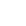My Wallet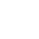My Order
•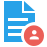My Profile
•My Connections
•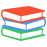My Books
•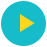My Videos
•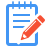My Tests
•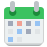My Calender
•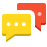My Messages
•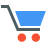My Shopping Cart
•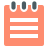My Orders
•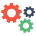Account Settings
•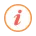Help

# Book Details# Operations Research (JNTU-K) R16

 Course Code : B.Tech IV-II (CSE) Author : SIA PUBLISHERS University : JNTU Kakinada Regulation : 2020 Categories : Engineering Format :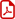PDF (DRM Protected) Type : eBook

Rs.109

Description :

SYLLABUS [1-6 UNITS]

UNIT-I:

Introduction to Operations Research: Basics definition, scope, objectives, phases, models and limitations of Operations Research. Linear Programming Problem -- Formulation of LPP, Graphical solution of LPP. Simplex Method, Artificial variables, big-M method, two-phase method, degeneracy and unbound solutions.

UNIT-II:

Transportation Problem. Formulation, solution, unbalanced Transportation problem. Finding basic feasible solutions -- Northwest corner rule, least cost method and Vogel’s approximation method. Optimality test: the stepping stone method and MODI method

UNIT-III:

Assignment model. Formulation. Hungarian method for optimal solution. Solving unbalanced problem. Traveling salesman problem and assignment problem Sequencing models. Solution of Sequencing Problem -- Processing n Jobs through 2 Machines -- Processing n Jobs through 3 Machines -- Processing 2 Jobs through m machines -- Processing n Jobs through m Machines

UNIT-IV:

Dynamic programming. Characteristics of dynamic programming. Dynamic programming approach for Priority Management employment smoothening, capital budgeting, Stage Coach/Shortest Path, cargo loading and Reliability problems Games Theory. Competitive games, rectangular game, saddle point, minimax (maximin) method of optimal strategies, value of the game. Solution of games with saddle points, dominance principle. Rectangular games without saddle point -- mixed strategy for 2 X 2 games

UNIT-V:

Replacement Models. Replacement of Items that Deteriorate whose maintenance costs increase with time without change in the money value. Replacement of items that fail suddenly: individual replacement policy, group replacement policy

UNIT-VI:

Inventory models. Inventory costs. Modelswith deterministic demand -- model (a) demand rate uniform and production rate infinite, model (b) demand rate non-uniform and production rate infinite, model (c) demand rate uniform and production rate finite.

SYLLABUS [1-8 UNITS]

Unit-I

Introduction to operations research: Basic definitions, Scope, Objectives, Phases, Models and limitations of operations research. Linear programming problem – Formulation of LPP, Graphical solution of LLP. Simplex method, Artificial variables, Big-M method, Two-phase method, Degeneracy and unbound solutions.

Unit-II

Transportation problem. Formulation, Solution, Unbalanced transportation problem. Finding basic feasible solutions – Northwest corner rule, Least cost method and Vogel’s approximation method. Optimality test: The stepping stone method and MODI method.

Unit-III

Assignment model. Formulation. Hungarian method for optimal solution. Solving unbalanced problem. Traveling salesman problem and assignment problem.

Unit-IV

Sequencing models. Solution of sequencing problem – Processing n jobs through 2 machines – Processing n jobs through 3 machines – Processing 2 jobs through m machines – Processing n jobs through m machines.

Unit-V

Dynamic programming. Characteristics of dynamic programming. Dynamic programming approach for priority management, Employment smoothening, Capital budgeting, Stage coach/shortest path, Cargo loading and reliability problems.

Unit-VI

Games theory. Competitive games, Rectangular game, Saddle point, Minimax (maximin) method of optimal strategies, Value of the game. Solution of games with saddle points, Dominance principle. Rectangular games without saddle point – Mixed strategy for 2 X 2 games.

Unit-VII

Replacement models. Replacement of items that deteriorate whose maintenance costs increase with time without change in the money value. Replacement of items that fail suddenly: Individual replacement policy, Group replacement policy.

Unit-VIII

Inventory models. Inventory costs. Models with deterministic demand – Model (a) demand rate uniform and production rate infinite, Model (b) demand rate non-uniform and production rate infinite, Model (c) demand rate uniform and production rate finite.

Note : Operations Research, B.Tech JNTU-K CSE R16

##### Publisher Detail:

Publisher Name: SIA Publishers and Distributors (P) Ltd.

Contact Email Id: msultan@siaedugroup.com